# MacWilliams identities

(diff) ← Older revision | Latest revision (diff) | Newer revision → (diff)

A-dimensional subspaceof the linear spaceof all-tuples over the finite field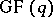is called an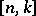linear code over. The vectors inare called code words. The weight of a vector is the number of its non-zero components. A generator matrix for a codeis any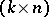-matrixwhose rows form a basis of. The ordinary inner product of vectors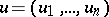,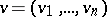inisThe dual ofis the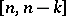linear code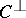defined by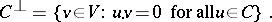The weight distribution of a codeof lengthgives the number of vectors of each weight,, denoted by. For example, letbe the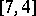linear code overwith generating matrix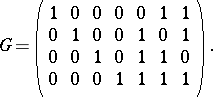The only non-zero weights inare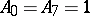,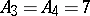.

While the weight distribution does not, in general, uniquely determine a code, it gives important information that has both practical and theoretical significance. The most fundamental result about weight distributions are the MacWilliams equations, a set of linear relations between the weight distributions ofand. These equations imply that the weight distribution ofis uniquely determined by the weight distribution of. These relations have been the most significant tool available for investigating and calculating weight distributions.

Let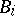denote the number of vectors of weightin. Ifis anlinear code over, then there are two equivalent sets of MacWilliams equations [a1]: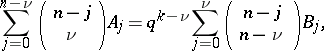(a1)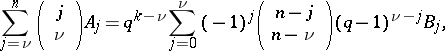(a2)

where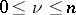.

If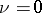, both sets of equations say that, which states the obvious result that ancode overcontains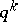codewords. The other equations are not so obvious. One can solve either set of equations for the weight distribution of, obtaining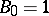and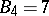.

An elegant and much used way to express these relations is in terms of polynomials. The weight enumerator of a codeof lengthis: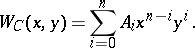The following equation is equivalent to either (a1) or (a2) for a codeover: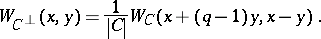(a3)

Two other sets of equations equivalent to either (a1), (a2) or (a3) and more convenient for some calculations are called the power moment identities [a3]. They involve the Stirling numbers of the second kind(cf. also Combinatorial analysis). One such set is(a4)where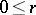.

The power moments are particularly useful for giving conditions under which the weight distributions of a code and its dual are uniquely determined. This is illustrated in the following theorem: A unique solution to the power moments exists under the conditions thator fewer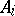's are unknown and that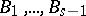are known [a3]. In this situation, the weight distributions of bothandare uniquely determined.

There are also MacWilliams equations for non-linear codes [a2].

How to Cite This Entry:
MacWilliams identities. Encyclopedia of Mathematics. URL: http://encyclopediaofmath.org/index.php?title=MacWilliams_identities&oldid=16837
This article was adapted from an original article by V. Pless (originator), which appeared in Encyclopedia of Mathematics - ISBN 1402006098. See original article